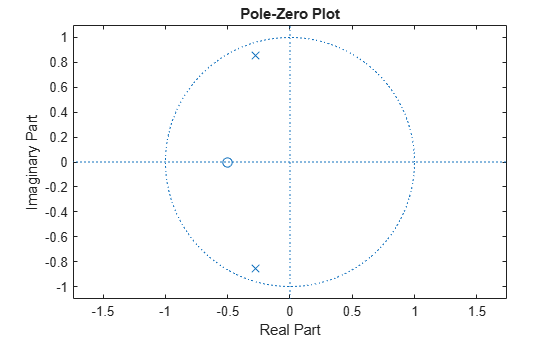Documentation

Zero-Pole Analysis

The zplane function plots poles and zeros of a linear system. For example, a simple filter with a zero at -1/2 and a complex pole pair at $0.9{e}^{-j2\pi 0.3}$ and $0.9{e}^{j2\pi 0.3}$ is

zer = -0.5;
pol = 0.9*exp(j*2*pi*[-0.3 0.3]');

To view the pole-zero plot for this filter you can use zplane. Supply column vector arguments when the system is in pole-zero form.

zplane(zer,pol)For access to additional tools, use fvtool. First convert the poles and zeros to transfer function form, then call fvtool.

[b,a] = zp2tf(zer,pol,1);
fvtool(b,a)Click the Pole/Zero Plot toolbar button, select Analysis > Pole/Zero Plot from the menu, or type the following code to see the plot.

fvtool(b,a,'Analysis','polezero')To use zplane for a system in transfer function form, supply row vector arguments. In this case, zplane finds the roots of the numerator and denominator using the roots function and plots the resulting zeros and poles.

zplane(b,a)See Discrete-Time System Models for details on zero-pole and transfer function representation of systems.